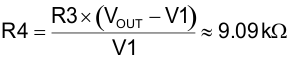SLUS157Q December   1999  – October 2019

PRODUCTION DATA.

1. Features
2. Applications
3. Description
1.     Device Images
4. Revision History
5. Pin Configuration and Functions
6. Specifications
7. Detailed Description
8. Application and Implementation
1. 8.1 Application Information
2. 8.2 Typical Application
1. 8.2.1 Design Requirements
2. 8.2.2 Detailed Design Procedure
3. 8.2.3 Application Curves
9. Power Supply Recommendations
10. 10Layout
11. 11Device and Documentation Support
12. 12Mechanical, Packaging, and Orderable Information

• DW|20
• PW|20
• N|20
• PW|20

#### 8.2.2.10 Current Sense Network (CT, RCS, RR, DA)

The CT chosen for this design has a turns ratio (CTRAT) of 100:1.

Equation 82.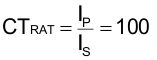Calculate nominal peak current (IP1) at VINMIN:

Peak primary current:

Equation 83.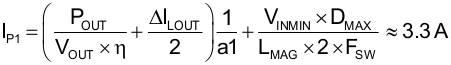The CS pin voltage where peak current limit will trip.

Equation 84.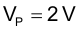Calculate current sense resistor (RCS) and leave 300 mV for slope compensation. Include a 1.1 factor for margin:

Equation 85.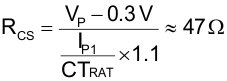Select a standard resistor for RCS:

Equation 86.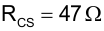Estimate power loss for RCS:

Equation 87.Calculate maximum reverse voltage (VDA) on DA:

Equation 88.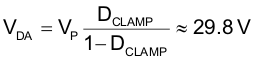Estimate DA power loss (PDA):

Equation 89.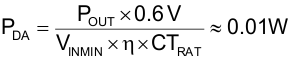Calculate reset resistor RR:

Resistor RR is used to reset the current sense transformer CT.

Equation 90.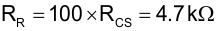Resistor RLF and capacitor CLF form a low pass filter for the current sense signal (Pin 15). For this design we chose the following values. This filter has a low frequency pole (fLFP) at 482 kHz. This should work for most applications but may be adjusted to suit individual layouts and EMI present in the design.

Equation 91.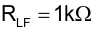Equation 92.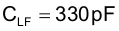Equation 93.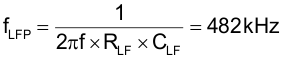The UCC3895 REF output (Pin 4) needs a high frequency bypass capacitor to filter out high frequency noise. The maximum amount of capacitance allowed is given in the Recommended Operating Conditions.

Equation 94.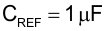The voltage amplifier reference voltage (Pin 2, EA+) can be set with a voltage divider (R1, R2), for this design example, the error amplifier reference voltage (V1) will be set to 2.5 V. Select a standard resistor value for R1 and then calculate resistor value R2.

UCC3895 reference voltage:

Equation 95.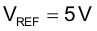Set voltage amplifier reference voltage:

Equation 96.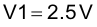Equation 97.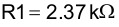Equation 98.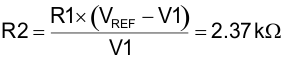Voltage divider formed by resistor R3 and R4 are chosen to set the DC output voltage (VOUT) at Pin 3 (EA–).

Select a standard resistor for R3:

Equation 99.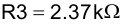Calculate R4:

Equation 100.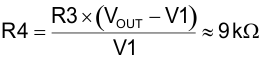Then choose a standard resistor for R4:

Equation 101.